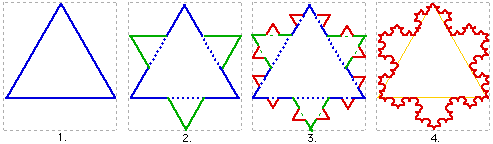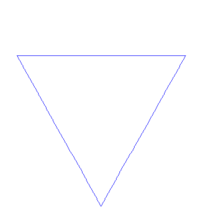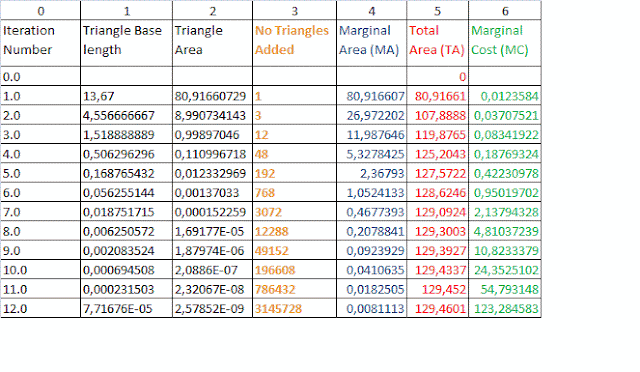## Sunday, January 30, 2011

### Fractal equilibrium count

Continuing on from my earlier blog on fractal equilibrium:Koch Curve Animation
From a fixed view point: all fractals ('attractors')  form their shape (are at fractal equilibrium) at and around 7 plus or minus 2  iterations - any more than this will come at too high a cost, and with no extra benefit - as shown in the animation of the Koch Snowflake development above.
The 5 iterations to develop the fractal Koch snowflake in fig. 1 (below) - the point where the blue extra (Marginal) area (MA) and green extra (Marginal) cost (MC) intersect, corresponds with where the shape of the snowflake is fully developed.
This, I believe, is not only a demonstration but is an explanation for  The Magical Number - Seven, Plus or Minus Two, but is also observable through-out our reality.

From any stand-point, there will be around 4,5,6,7,or 8 levels of protrusion. For example, from where I am writing, I can see out my window where there is a park and some buildings. The building is the first protrusion, then there is a chimney on the building - 2, then a brick on the chimney -3, then there is, I can just see, an icicle (it is winter) -4.
See my entry on fractal paradigm

(http://www.musanim.com/miller1956/ )

In the image of a tree to the left, if you follow out from the trunk of the tree, until it first forks, then follow that branch until it forks again, and then go on repeating, following the 'first' branch on the branch, until you cannot see any more branches, you will find you can only fork 7 + or - 2 times.

Iteration No 6 is the optimal or perfect viewing iteration of the (fractal) Koch Snowflake - from the viewers perspective.

I believe it is also the number of iterations, or feedback's, to gain market equilibrium.

The following cases are of interest to me:
• 6 degrees of separation - between knowing everybody on the earth: I have heard that the reality is around 4 before the link ends, fades away.
• How many times can one fold a piece of paper - 7, 8,9, maybe 13 - but not more (??)
• The food-chain: How many links between the top and the bottom of the food-chain. With whales and plankton, not many, but I have been told the max is 6. After that there is nothing to be gained, and what is comes at too higher cost.

### Fractal: Equilibrium Perfect Knowledge and Output

Fractal Equilibrium:
This entry follows on from 'production of the fractal'.
Here I am suggesting that equilibrium - in any sense - is a fundamental of the fractal.Koch Snowflake development: source, Wikipedia

The above animation shows the development of the fractal, at iteration 5 or 6 fractal equilibrium is reached - where the shape (of the snowflake) is made; or where benefit production is equal to cost of production.

Fig. 2b below, shows a closeup analysis of the fractal equilibrium, at least from a static point of view.  MC  intersects, or is equal to MA, at iteration 5 where the Area is equal to 1, due to the reciprocal of 1 itself being equal 1.

Equilibrium - Perfect Knowledge*
http://en.wikipedia.org/wiki/Perfect_information
Any iteration less than fractal equilibrium will result in an imperfect (snowflake) shape or incomplete knowledge or information.
Any iteration point greater fractal equilibrium will result with little added gain in information or shape -  and it will come at a great cost.
Perfect knowledge, or the perfect shape, is gained where MA =MC.  This, it taken directly from  economics theory, resembles where allocative efficiency is maximized - at the equilibrium point in market theory. Competition gives the best shape or knowledge, opposed to a monopolist that would produce or output somewhere left of equilibrium.
Notice Total knowledge will never be gained - the cost is too high.
*I am aware that the fractal demonstrates infinity, and thus shows the infinity of knowledge - of the rule. To say perfect information may well be a relative statement, said in context, or in a  paradigm.

A theory of knowing?
Development of the little man is my attempt at understanding how we know: check out my theory of mind

 http://www.fractalnomics.com/2011/09/paradigm-fractal.htmlEquilibrium Output (quantity)
Of course, iteration number is not the output or quantity ( as in classical economics). So, what then is it?
It came to me that it is the number of triangles - that are added after each iteration.
In the below diagrams below (fig.3 and 3b), shown in orange is the increase in the quantity of triangles, after each iteration. I have recently learned that this curve is exponential.

Equilibrium quantity or output at fractal equilibrium is  ..... triangles or point 'a', on the diagram.
Update May 2017
This is by far my best idea; I have written it up in a working paper at my academia.edu and vixra, and named it:  Quantum Mechanics, Information and Knowledge, all Aspects of Fractal Geometry and Revealed in an Understanding of Marginal Economics.
I shall post the Abstract, followed by the original post, followed by the paper. I hope to have some collaborate and review my work in time.
Abstract
Fractal geometry is found universally and is said to be one of the best descriptions of our reality – from clouds and trees, to market price behaviour. As a fractal structure emerges – the repeating of a simple rule – it appears to share direct properties familiar to classical economics, including production, consumption, and equilibrium. This paper was an investigation into whether the mathematical principles behind ‘the market’ – known as marginalism – is an aspect or manifestation of a fractal geometry or attractor. Total and marginal areas (assumed to stand for utility) and the cost of production were graphed as the fractal grew and compared to a classical interpretation of diminishing marginal utility theory, and the market supply and demand. PED and PES was also calculated and analysed with respect to (iteration) time and decay.  It was found the fractal attractor demonstrates properties and best models classical economic theory and from this it was deduced the market is a fractal attractor phenomenon where all properties are inextricably linked. The fractal, at equilibrium, appears to be a convergent – zeta function – series, able to be described by Fourier analysis, and involves Pi, i, e, 0, and 1 (of Euler’s identity) in one model. It also demonstrated growth, development, evolution and Say’s Law – production before consumption. Insights from the fractal on knowledge and knowing are also revealed, with implications on the question of what exactly is ‘science’ – and what is ‘art’? A connect between reality and quantum mechanics was identified. It was concluded marginal, classical economics is an aspect of a fractal geometry.
Keywords
Marginal, Fractal, Elasticity, Utility, Cost, Production, Price, Growth and Development, Say’s Law

## Saturday, January 29, 2011

### Marginal Cost - Production of the Fractal

Marginal Cost - derived from the fractal

Original Post
The fractal demonstrates cost.
To demonstrate the increasing cost - in effort and time - at each iteration (see my first blog on marginal analysis and the fractal) to produce the fractal, I decided to use the the reciprocal and invert the Marginal Area, MA. The rationale for this choice is based on: the more area to the snowflake, the more the cost. I am sure there are other ways of doing this, but I figured that this method is as simple and as easy as calculating the MC again, it should be okay.
Fig. 2 shows the original MA TA diagram with the MC.

Background
One thing about computer generated fractals is that they appear to be produced with ease, we simply forget that they would not be viewable if it were not for the memory and processing power of our modern computer. To see how complex and slow they are to draw one only has to take a pen and paper and have a go at drawing the Koch snowflake progression - step by step. It is easy from the beginning, but it progressively gets harder and harder with each iteration or level: there is a 'cost' in time, and it takes patience too - adding hundreds of triangles and rubbing out old lines.
What we don't see when we use the modern computer to draw them is that they too will start to labour, and slow - after (around) the 8th iteration, and probably crash after the 12th . This all due to the added complexity at each iteration.

In my next entry I will analyse this fractal equilibrium.
Figure 2b shows the MC MA equilibrium in closeup.

Update May 2017
This is by far my best idea; I have written it up in a working paper at my academia.edu and vixra, and named it:  Quantum Mechanics, Information and Knowledge, all Aspects of Fractal Geometry and Revealed in an Understanding of Marginal Economics.
I shall post the Abstract, followed by the original post, followed by the paper. I hope to have some collaborate and review my work in time.
Abstract
Fractal geometry is found universally and is said to be one of the best descriptions of our reality – from clouds and trees, to market price behaviour. As a fractal structure emerges – the repeating of a simple rule – it appears to share direct properties familiar to classical economics, including production, consumption, and equilibrium. This paper was an investigation into whether the mathematical principles behind ‘the market’ – known as marginalism – is an aspect or manifestation of a fractal geometry or attractor. Total and marginal areas (assumed to stand for utility) and the cost of production were graphed as the fractal grew and compared to a classical interpretation of diminishing marginal utility theory, and the market supply and demand. PED and PES was also calculated and analysed with respect to (iteration) time and decay.  It was found the fractal attractor demonstrates properties and best models classical economic theory and from this it was deduced the market is a fractal attractor phenomenon where all properties are inextricably linked. The fractal, at equilibrium, appears to be a convergent – zeta function – series, able to be described by Fourier analysis, and involves Pi, i, e, 0, and 1 (of Euler’s identity) in one model. It also demonstrated growth, development, evolution and Say’s Law – production before consumption. Insights from the fractal on knowledge and knowing are also revealed, with implications on the question of what exactly is ‘science’ – and what is ‘art’? A connect between reality and quantum mechanics was identified. It was concluded marginal, classical economics is an aspect of a fractal geometry.
Keywords
Marginal, Fractal, Elasticity, Utility, Cost, Production, Price, Growth and Development, Say’s Law

## Thursday, January 27, 2011

### Marginal Analysis of the Fractal

Marginalism and Marginal Analysis : derived from the fractal.
Here I preform basic marginal analysis on the Koch Snowflake
Update May 2017
This is by far my best idea; I have written it up in a working paper at my academia.edu and vixra, and named it:  Quantum Mechanics, Information and Knowledge, all Aspects of Fractal Geometry and Revealed in an Understanding of Marginal Economics.
I shall post the Abstract, followed by the original post. I hope to have some collaborate and review my work in time.

Abstract
Fractal geometry is found universally and is said to be one of the best descriptions of our reality – from clouds and trees, to market price behaviour. As a fractal structure emerges – the repeating of a simple rule – it appears to share direct properties familiar to classical economics, including production, consumption, and equilibrium. This paper was an investigation into whether the mathematical principles behind ‘the market’ – known as marginalism – is an aspect or manifestation of a fractal geometry or attractor. Total and marginal areas (assumed to stand for utility) and the cost of production were graphed as the fractal grew and compared to a classical interpretation of diminishing marginal utility theory, and the market supply and demand. PED and PES was also calculated and analysed with respect to (iteration) time and decay.  It was found the fractal attractor demonstrates properties and best models classical economic theory and from this it was deduced the market is a fractal attractor phenomenon where all properties are inextricably linked. The fractal, at equilibrium, appears to be a convergent – zeta function – series, able to be described by Fourier analysis, and involves Pi, i, e, 0, and 1 (of Euler’s identity) in one model. It also demonstrated growth, development, evolution and Say’s Law – production before consumption. Insights from the fractal on knowledge and knowing are also revealed, with implications on the question of what exactly is ‘science’ – and what is ‘art’? A connect between reality and quantum mechanics was identified. It was concluded marginal, classical economics is an aspect of a fractal geometry.
Keywords
Marginal, Fractal, Elasticity, Utility, Cost, Production, Price, Growth and Development, Say’s Law

Intro
For some time now, I have pondered on how the Koch Snowflake fractal (seen below) demonstrates, uncannily, key features in economic theory.
Is it that the economy - as we experience it - is a fractal? or, is the fractal an economy?
I am now confident - after doing the following basic math's - that fractal geometry is the foundation to the social science, Economics.Koch Snowflake animation:

Towards Equilibrium
From the outset, the concept of Marginalism and fractals have something in common, they are both about the next -the next unit, or the next iteration.

Once a rule, in this case new triangles,  is set in action and allowed to progress (iterate) step by step, it will go on to eventually form a snowflake.  After iteration 4, the shape will set and no longer change - at least from the viewers' static perspective.Koch Snowflake development

If one were to continue the iterations beyond the point of equilibrium (4.) the computer generating the pattern would soon crash:  the effort (cost) would multiply while the extra benefit to the viewer - in terms of the shape – would fall close to, but never, zero.
Take the time to look at this webpage http://mathforum.org/mathimages/index.php/Koch_Curve where you can experience the fill working of the Koch Curve first hand.

Method.
I analysed the growth of the Koch Snowflake using marginal analysis (a method found in any basic economic textbook) which looks at (in this case) the extra 'area' added - iteration by iteration.

Working from internet sources I constructed a table showing the area progression of the curve - iteration after iteration:

Taking s as the side length, the original triangle area is . The side length of each successive small triangle is 1/3 of those in the previous iteration; because the area of the added triangles is proportional to the square of its side length, the area of each triangle added in the nth step is 1/9th of that in the (n-1)th step. In each iteration after the first, 4 times as many triangles are added as in the previous iteration; because the first iteration adds 3 triangles, the nth iteration will add triangles. Combining these two formulae gives the iteration formula:

Area (A) of each triangle (as in column 2) = (s^2 √(3))/4

To show that the fractal Koch Snow Flake demonstrates marginal utility theory, I substituted the Economists' utility (satisfaction or benefit gained from another iteration) with the area of the curve: reasoning that after more iterations, one gets more total satisfaction or in this case Total Area (TA). One gets (pleasingly) closer to the true shape of the snowflake.
I have used no units to measure Area, as the area in the diagram corresponds to a curve I used as a reference, http://math.rice.edu/~lanius/frac/koch3.html on the internet.

Results Table

This result table will be central to my analysis of the fractal.

## Saturday, January 8, 2011

### Lorenz Curve of the Koch Snowflake Fractal

Update 2015, I have published/posted.
I have found; Lorenz distribution is a fractal phenomenon;  the fractal models the Lorenz Curve; Gini coefficients increase as the fractal grows and develops; and the distribution between groups accelerates with growth and development. Lorenz distribution is universal: income and wealth inequality one aspect of a universal phenomenon, and is scale invariant.

Wealth Distribution: a (universal) fractal phenomena
I was teaching income distribution recently, and I thought maybe the (Koch Curve) fractal demonstrates the Lorenz Curve.
After doing this entry I analysed a (Xmas) tree for Lorenz distribution. Click to see.

I could not make any obvious connection between the Lorenz Curve's income distribution and the Koch showflake development: maybe because income is a flow concept and the Koch snowflake doesn't seem to demonstrate this.  So I thought: what distribution does it demonstrate? I turned my attention to the concept of wealth distribution. I assumed that Wealth corresponds better with the Area  - reasoning that both are a 'stock' concept.
I did the calculations (taken from the area calculation table in my first blog 1.1 seen below) : percent of number of triangles, and percent of area for each triangle -and then corrected the results so as to view like the original Lorenz Curve.Koch Snowflake (fractal) development

ResultsAnalysis of the Koch SnowflakeLorenz Curve calculation

Diagram:Lorenz Curve for the Koch Snowflake
Thinking that this Lorenz Curve was rather exaggerated to the right - with a very large Gini coefficient  - I searched for a reference to agree with this extreme position - in 'nature' or the real world. I found the following wealth Lorenz Curve reference for the USA economy in one of my economics texts.Lorenz Curve from Book: Economics, Micheal Parkin, Addison-Wesley 1990

Discussions and Conclusions
The wealth and income  Lorenz Curves are a fractal phenomena and is best demonstrated using the (Koch Snowflake) fractal.
Lorenz distribution is a universal phenomena, inherent in all systems fractal and at all scales. Income and wealth distribution are just two manifestations of this structure and thus is not directly determined by Economic output or growth - they are 'natural'.
I look forward to making real Lorenz curves from real fractals: trees, rivers, and the like.

2011-01-15
BBC article on Wikipedia:
Listening to the below podcast, I heard a reference made to the concept of distribution of article posting - most of the articles are added by a very few.

2012-12-10
It interested me when I saw this image on facebook: why poverty now :

My mind immediately saw the Fibonacci resemblance.